# m Derived High Pass Filter

## m Derived High Pass Filter:

The m Derived High Pass Filter T and π sections are as shown in the Fig. 9.32 (a) and (b).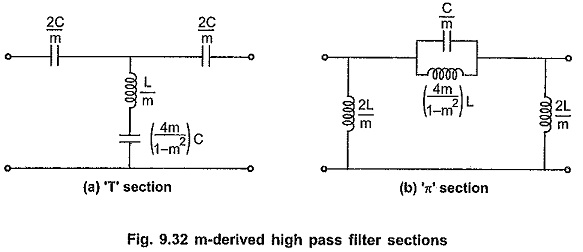Consider that the shunt arm of the T section resonates at a frequency of infinite attenuation i.e. f which is selected just below cut-off frequency fc. The frequency of resonance is given by,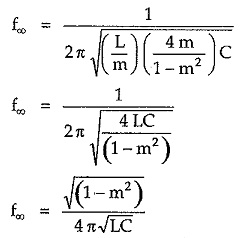But for high pass filter, cut-off frequency is given by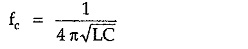Substituting value of fc in the equation for f,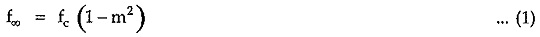Simplifying equation (1) further,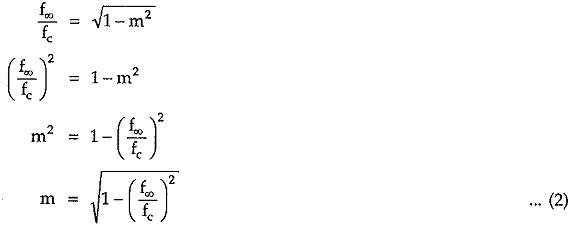#### Variations of Characteristic Impedance (Z0), Attenuation Constant (α) and Phase Shift (β) with Frequency:

The variations of characteristic impedance (Z0), attenuation constant (α) and phase shift (β) with frequency are as shown in the Fig. 9.33 (a), (b) and (c) respectively.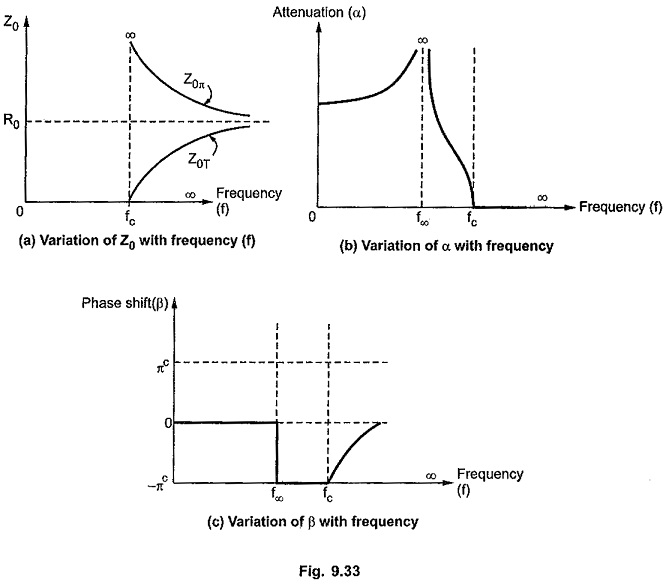As the characteristic impedance for both the sections i.e. prototype section and m Derived High Pass Filter section, the variation of Z0 in m-derived section is similar to that in the prototype filter section.

The attenuation constant α in the attenuation band is given by,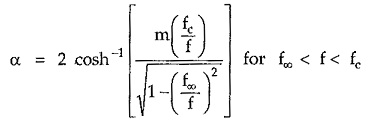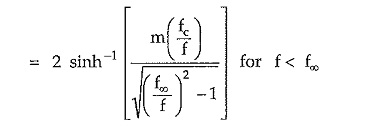The phase shift β in the pass band is given by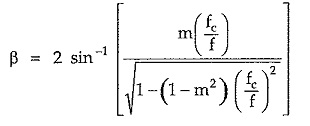Updated: November 13, 2019 — 9:38 pm